# Analyze Data in Multivariable Systems and Identify Models

This example shows how to deal with data with several input and output channels (MIMO data). Common operations, such as viewing the MIMO data, estimating and comparing models, and viewing the corresponding model responses are highlighted.

### The Data Set

We start by looking at the data set SteamEng.

`load SteamEng`

This data set is collected from a laboratory scale steam engine. It has the inputs Pressure of the steam (actually compressed air) after the control valve, and Magnetization voltage over the generator connected to the output axis.

The outputs are Generated voltage in the generator and Rotational speed of the generator (Frequency of the generated AC voltage).The sample time was 50 ms.

First collect the measured channels into an `iddata` object:

```steam = iddata([GenVolt,Speed],[Pressure,MagVolt],0.05); steam.InputName = {'Pressure';'MagVolt'}; steam.OutputName = {'GenVolt';'Speed'};```

Let us have a look at the data

`plot(steam(:,1,1))`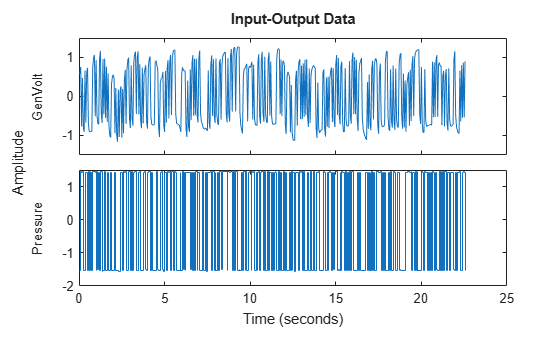`plot(steam(:,1,2))`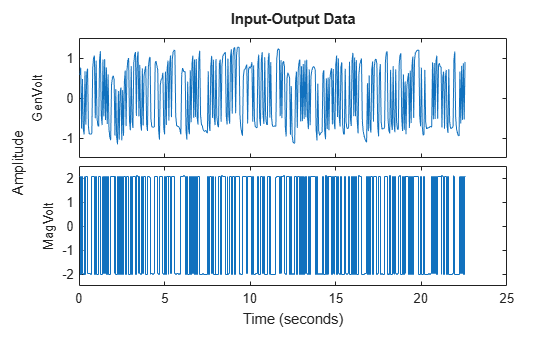`plot(steam(:,2,1))``plot(steam(:,2,2))`### Step and Impulse Responses

A first step to get a feel for the dynamics is to look at the step responses between the different channels estimated directly from data:

```mi = impulseest(steam,50); clf, step(mi)```### Responses with Confidence Regions

To look at the significance of the responses, the impulse plot can be used instead, with confidence regions corresponding to 3 standard deviations:

`showConfidence(impulseplot(mi),3)`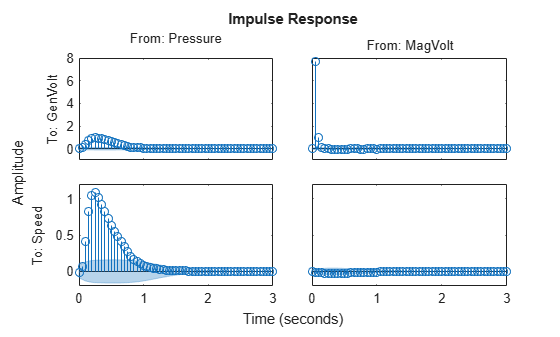Clearly the off-diagonal influences dominate (Compare the y-scales!) That is, `GenVolt` is primarily affected by `MagVolt` (not much dynamics) and `Speed` primarily depends on `Pressure`. Apparently the response from `MagVolt` to `Speed` is not very significant.

### A Two-Input-Two-Output Model

A quick first test is also to look a a default continuous time state-space prediction error model. Use only the first half of the data for estimation:

`mp = ssest(steam(1:250))`
```mp = Continuous-time identified state-space model: dx/dt = A x(t) + B u(t) + K e(t) y(t) = C x(t) + D u(t) + e(t) A = x1 x2 x3 x4 x1 -29.43 -4.561 0.5994 -5.2 x2 0.4849 -0.8662 -4.101 -2.336 x3 2.839 5.084 -8.566 -3.855 x4 -12.13 0.9224 1.818 -34.29 B = Pressure MagVolt x1 0.1033 -1.617 x2 -0.3027 -0.09415 x3 -1.566 0.2953 x4 -0.04477 -2.681 C = x1 x2 x3 x4 GenVolt -16.39 0.3767 -0.7566 2.808 Speed -5.623 2.246 -0.5356 3.423 D = Pressure MagVolt GenVolt 0 0 Speed 0 0 K = GenVolt Speed x1 -0.3555 0.0853 x2 -0.0231 5.195 x3 1.526 2.132 x4 1.787 0.03216 Parameterization: FREE form (all coefficients in A, B, C free). Feedthrough: none Disturbance component: estimate Number of free coefficients: 40 Use "idssdata", "getpvec", "getcov" for parameters and their uncertainties. Status: Estimated using SSEST on time domain data. Fit to estimation data: [86.9;74.84]% (prediction focus) FPE: 3.896e-05, MSE: 0.01414 ```

Compare with the step responses estimated directly from data:

```h = stepplot(mi,'b',mp,'r',2); % Blue for direct estimate, red for mp showConfidence(h)```The agreement is good with the variation permissible within the shown confidence bounds.

To test the quality of the state-space model, simulate it on the part of data that was not used for estimation and compare the outputs:

`compare(steam(251:450),mp)`The model is very good at reproducing the Generated Voltage for the validation data, and does a reasonable job also for the speed. (Use the pull-down menu to see the fits for the different outputs.)

### Spectral Analysis

Similarly, comparisons of the frequency response of `mp` with a spectral analysis estimate gives:

`msp = spa(steam);`

`bode(msp,mp)`

`clf, bode(msp,'b',mp,'r')`You can right-click on the plot and select the different I/O pairs for close looks. You can also choose 'Characteristics: Confidence Region' for a picture of the reliability of the bode plot.

As before the response from `MagVolt` to `Speed` is insignificant and difficult to estimate.

### Single-Input-Single-Output (SISO) Models

This data set quickly gave good models. Otherwise you often have to try out sub-models for certain channels, to see significant influences The toolbox objects give full support to the necessary bookkeeping in such work. The input and output names are central for this.

The step responses indicate that `MagVolt` primarily influences `GenVolt` while `Pressure` primarily affects `Speed`. Build two simple SISO model for this: Both names and numbers can be used when selecting channels.

```m1 = tfest(steam(1:250,'Speed','Pressure'),2,1); % TF model with 2 poles 1 zero m2 = tfest(steam(1:250,1,2),1,0) % Simple TF model with 1 pole.```
```m2 = From input "MagVolt" to output "GenVolt": 18.57 --------- s + 43.53 Continuous-time identified transfer function. Parameterization: Number of poles: 1 Number of zeros: 0 Number of free coefficients: 2 Use "tfdata", "getpvec", "getcov" for parameters and their uncertainties. Status: Estimated using TFEST on time domain data. Fit to estimation data: 73.34% FPE: 0.04645, MSE: 0.04535 ```

Compare these models with the MIMO model mp:

`compare(steam(251:450),m1,m2,mp)`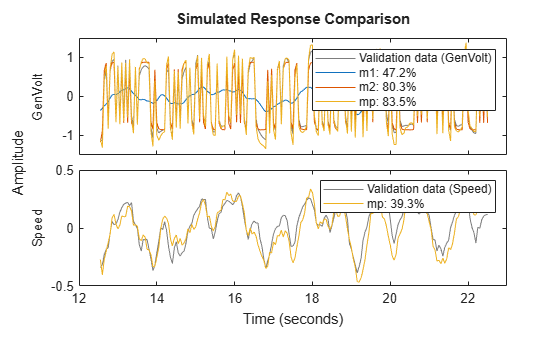The SISO models compare well with the full model. Let us now compare the Nyquist plots. `m1` is blue, `m2` is green and `mp` is red. Note that the sorting is automatic. `mp` describes all input output pairs, while `m1` only contains `Pressure` to `Speed` and `m2` only contains `MagVolt` to `GenVolt`.

```clf showConfidence(nyquistplot(m1,'b',m2,'g',mp,'r'),3)```The SISO models do a good job to reproduce their respective outputs.

The rule-of-thumb is that the model fitting becomes harder when you add more outputs (more to explain!) and simpler when you add more inputs.

### Two-Input-Single-Output Model

To do a good job on the output `GenVolt`, both inputs could be used.

```m3 = armax(steam(1:250,'GenVolt',:),'na',4,'nb',[4 4],'nc',2,'nk',[1 1]); m4 = tfest(steam(1:250,'GenVolt',:),2,1); compare(steam(251:450),mp,m3,m4,m2)```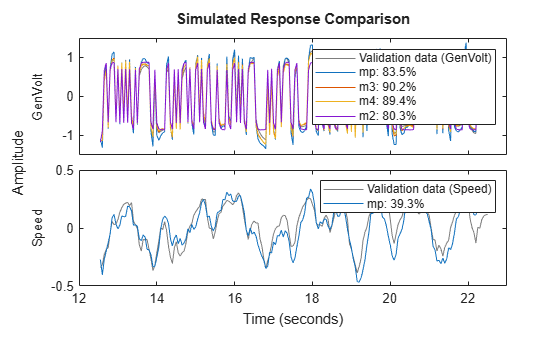About 10% improvement was possible by including the input `Pressure` in the models `m3` (discrete time) and `m4` (continuous time), compared to `m2` that uses just `MagVolt` as input.

### Merging SISO Models

If desired, the two SISO models `m1` and `m2` can be put together as one "Off-Diagonal" model by first creating a zero dummy model:

```mdum = idss(zeros(2,2),zeros(2,2),zeros(2,2),zeros(2,2)); mdum.InputName = steam.InputName; mdum.OutputName = steam.OutputName; mdum.ts = 0; % Continuous time model m12 = [idss(m1),mdum('Speed','MagVolt')]; % Adding Inputs. % From both inputs to Speed m22 = [mdum('GenVolt','Pressure'),idss(m2)]; % Adding Inputs. % From both inputs to GenVolt mm = [m12;m22]; % Adding the outputs to a 2-by-2 model. compare(steam(251:450),mp,mm)```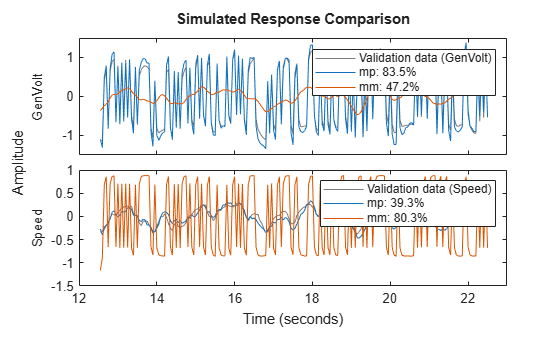Clearly the "Off-Diagonal" model `mm` performs like `m1` and `m2` in explaining the outputs.